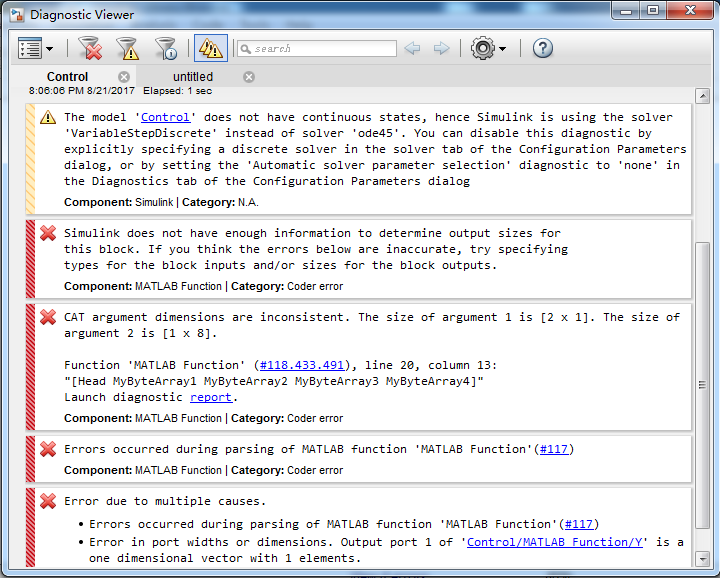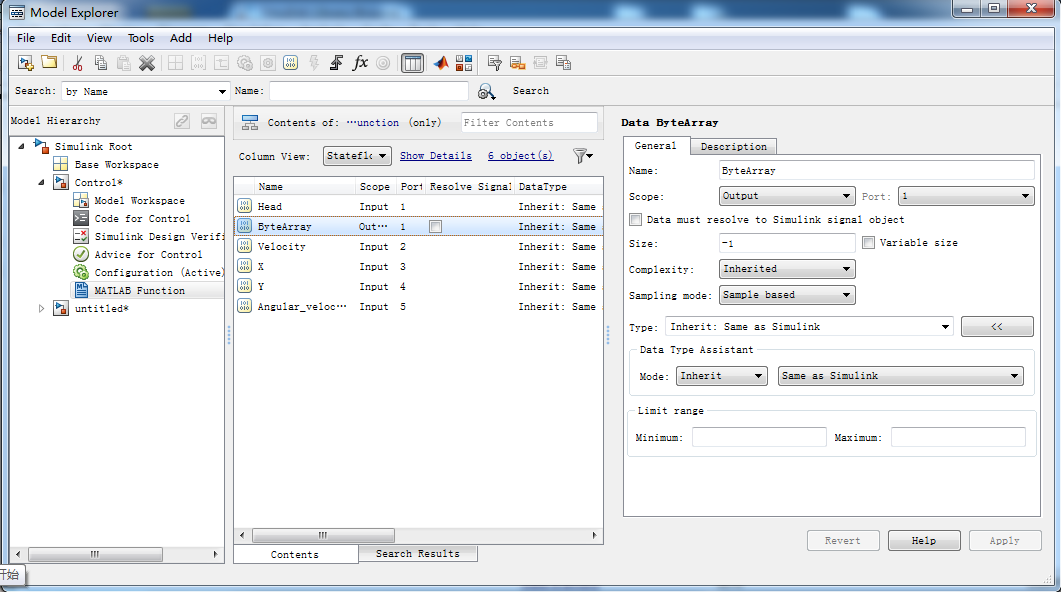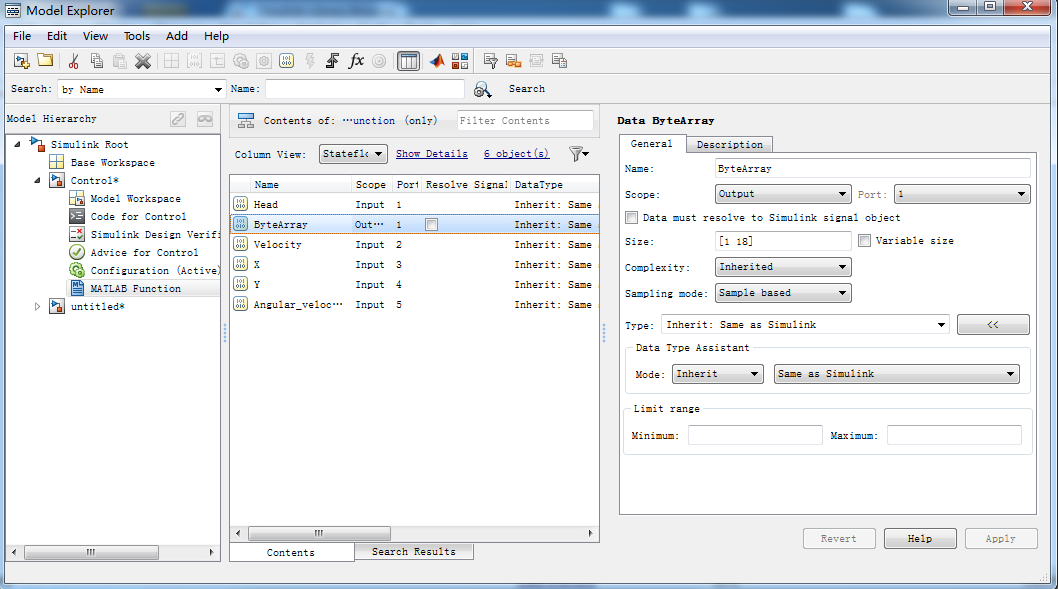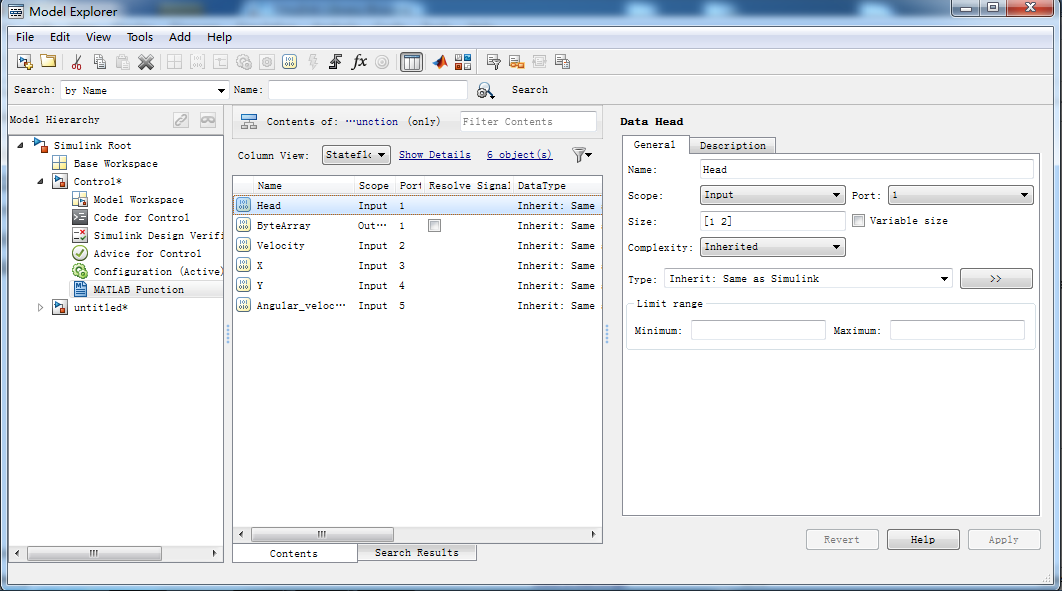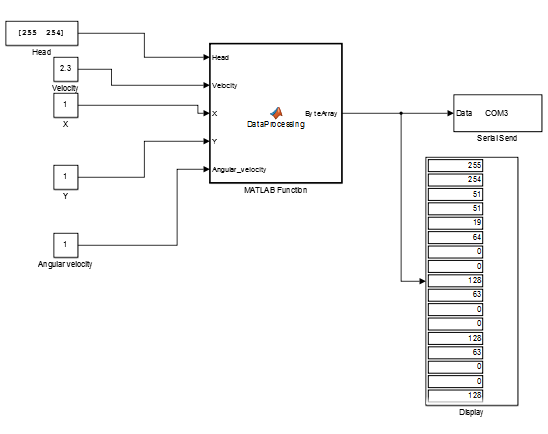# Matlab Simulink 串口通讯之float数据到字节数组（uint8）的相互转化

（1）数据类型与字节数组之间的相互转化

（2）.m文件中的方法：使用MATLAB中的typecast方法

1.将字节数组转化为float型数据

MyByteArray = [1 2 3 4];
MyByteArray = uint8(MyByteArray);
MySingle = typecast(MyByteArray,'single');

2.将float型数据转化为对应的uint8数组，也就是字节数组。

MySingle=single(3.24);
MyByteArray=typecast(MySingle,'uint8');

（3）Simulink中的方法:使用Simulink->MATLAB Function模块，在Matlab Function 模块中调用typecast函数，下面的例子将四个float型的数据转化为字节数组，加上数据的开始位，通过串口通讯发送到COM3。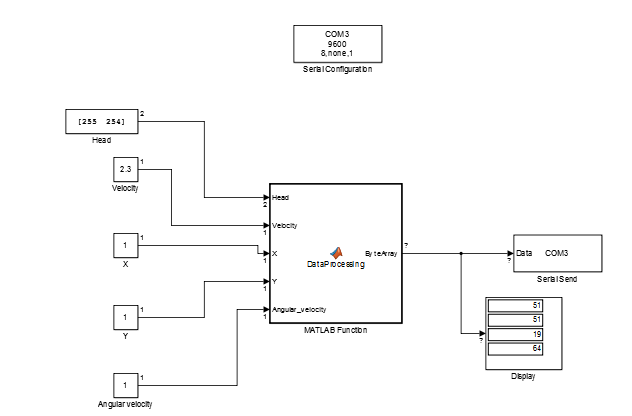Data Processing 函数：

function ByteArray = DataProcessing(Head,Velocity,X,Y,Angular_velocity)
Head=uint8(Head);
Velocity=single(Velocity);
X=single(X);
Y=single(Y);
Angular_velocity=single(Angular_velocity);

MyByteArray1=typecast(Velocity,'uint8');
MyByteArray2=typecast(X,'uint8');
MyByteArray3=typecast(Y,'uint8');
MyByteArray4=typecast(Angular_velocity,'uint8');

ByteArray = [Head MyByteArray1 MyByteArray2 MyByteArray3 MyByteArray4];